# SSC CGL EXAMS 2018 | Reasoning Ability Practice Questions (Day-12)

Dear Aspirants, Here we have given the Important SSC Exam 2018 Practice Test Papers. Candidates those who are preparing for SSC 2018 can practice these questions to get more confidence to Crack SSC 2018 Examination.

[WpProQuiz 4311]

1) In the following questions, find the old word/ letter/ number pair from the given alternatives.

b) Table Tennis

c) Cricket

d) Hockey

2) In the following questions, find the old word/ letter/ number pair from the given alternatives.

a) ACFJ

b) RTWA

c) NPSV

d) HJMQ

3)  In the following questions, find the old word/ letter/ number pair from the given alternatives.

a) (4, 16, 48)

b) (6, 36, 90)

c) (8, 64, 160)

d) (12, 144, 360)

4) Arrange the given words in the sequence in which they occur in the dictionary.

Decollate

Desecrate

Decorous

Despicable

Destitute

a) ACBDE

b) AEBDC

c) CBDEA

d) DEBCA

5) In the following question, which one set of letters when sequentially placed at the gaps in the given letter series shall complete it?

a_bc_a_bcdabc_da_cd_

a) acbdab

c) cabddc

d) ddcbbc

6) 5 yr hence, ratio of ages of A and B will be 7 : 5 and difference between their ages will be 4 yr. What are present ages (in yr) of A and B respectively?

a) 5 yr, 9 yr

b) 6 yr, 5 yr

c) 9 yr, 5 yr

d) 9 yr, 5 yr

7) From a point, Lokesh starts waking towards south and after waking 30 m he turns to his right and walks 20 m, and then he turns right again and walks 30 m. He finally turns to his left and walk 40 m. In which direction is he with reference to the starting point?

a) North – West

b) East

c) West

d) South

8) In a certain code language, ‘RAIN’ is written as ‘OHBQ’. How is ‘SUMMER’ written in that code language?

a) QFLNTT

b) QDLLTR

c) SFNNVT

d) SDNLVR

9) In the following question, correct the given equation by interchanging two numbers.

a) 3 and 4

b) 4 and 8

c) 5 and 3

d) 5 and 9

10) If (b)# *4 = 2 and (d)# *16, then what is the value of A in (6)# *A = 18?

a) 12

b) 14

c) 16

d) 20

11) In the following question, select the number which can be placed at the sign of question mark (?) from the given alternatives.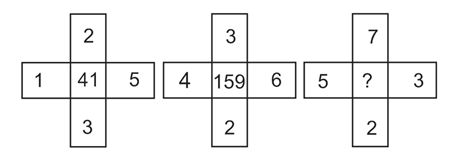12) In the following question below are given some statements followed by some conclusions. Taking the given statements to be true even if they seem to be at variance from commonly known facts, read all the conclusions and then decide which of the given conclusion logically follows the given statements?

Statements:     I.  All rackets are bats.

II.  All bats are wickets.

Conclusions:      I.  Some wickets are rackets.

II. All wickets are rackets.

13) From the given options, which answer the given options, which answer figure can be formed by folding the figure given in the question?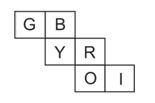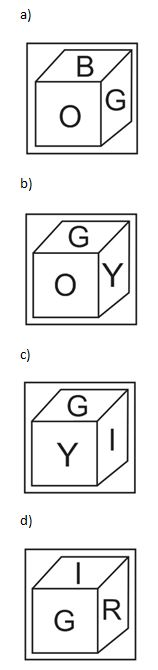14) Identify the diagram that best represents the relationship among the given classes.

Staff, Manager, Worker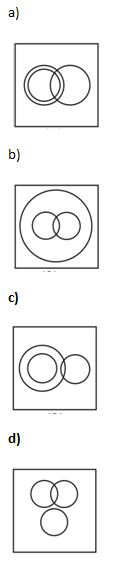15) From the given answer figures, select the one is which the question figure is hidden/ embedded.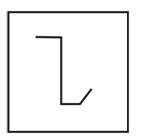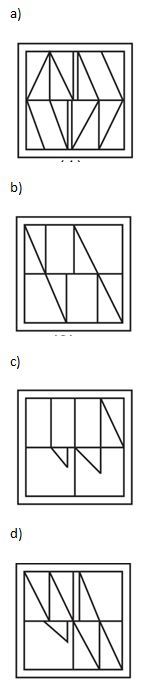Except Badminton, in all other games, ball is used to play. But Shuttle is used to play badminton.

As,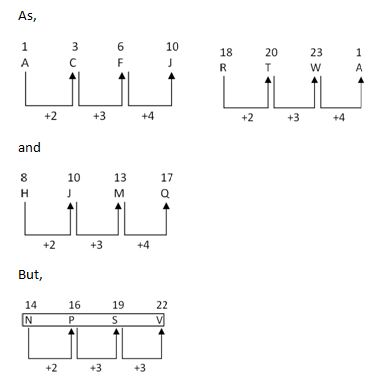and

But,

Hence, NPSV is different from others.

As,

(6)2 = 36 = 36 × (5/2) = 90

(8)2 = 64 = 64 × (5/2) = 160

(12)2 = 144 = 144 × (5/2) = 360

But, (4)2 = 16 = 16 × (5/2) = 20 ≠ 48

Hence, (4, 16, 48) is different from others.

Dictionary order is as follows,

Decollate =>  Decorous =>  Desecrate =>  Despicable =>  Destitute

1                      3                       2               4                        5

Dictionary order = 1, 3, 2, 4, 5

Order of series is as follows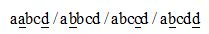Set adbcbd will complete the series when placed sequentially.

A’s present age = (7x – 5) yr

B’s present age = (5x – 5) yr

According to the question,

7x – 5 – 5x + 5 = 4

2x = 4

x = 2

A’s present age = 7×2 – 5 = 9 yr

B’s present age = 5×2 – 5 = 5 yr

Movement of Lokesh is as follows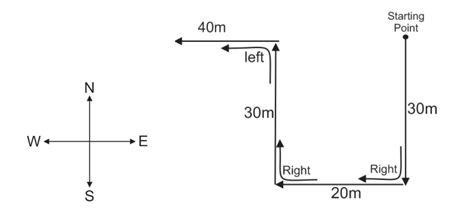Lokesh is in West direction from his starting point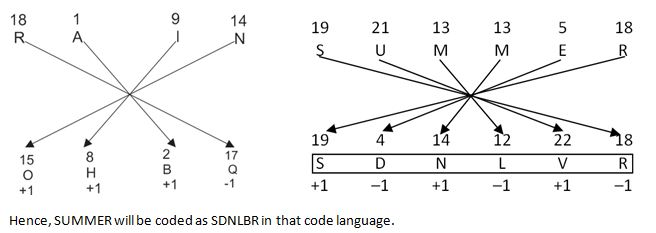Given equation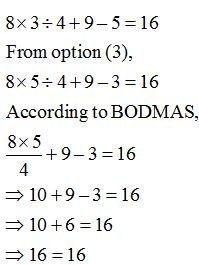As, (b)3  4 =23  4 = 8/4 = 2

(d)3  4 = 43 4 = 64 ÷ 4 = 16

Similarly, (6)3  A = 18

A = 216 ÷ 18 = 12

As, (1× 2 × 5 × 3) + (1 + 2 + 5 + 3)

= 30 + 11 = 41

(4 × 3 × 6 × 2) + (4 + 3 + 6 + 2)

= 210 + 17 =227

= 144 + 15 = 159

Similarly, (5  7  3  2) + (5 + 7 + 3 + 2)

= 210 + 17 = 227

According to statements,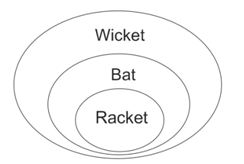Hence, only conclusion I follow.

Question FigureOpposite Surface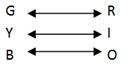Opposite surface can never be seen in a cube. Hence, only answer figure (a) can be form from the question figure.

Venn diagram is as follows.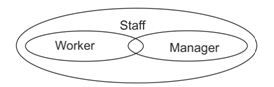Question figure is embedded in answer figure (a).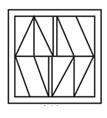#### *********************************